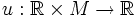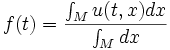# Timewise-average function

Let$M$ be a differential manifold equipped with a measure over which we can integrate.
Let$u:\R \times M \to \R$ be any continuous function. Then the timewise-average function of$u$ is a map$f:\R \to \R$ defined by:$f(t) = \frac{\int_M u(t,x) dx}{\int_M dx}$